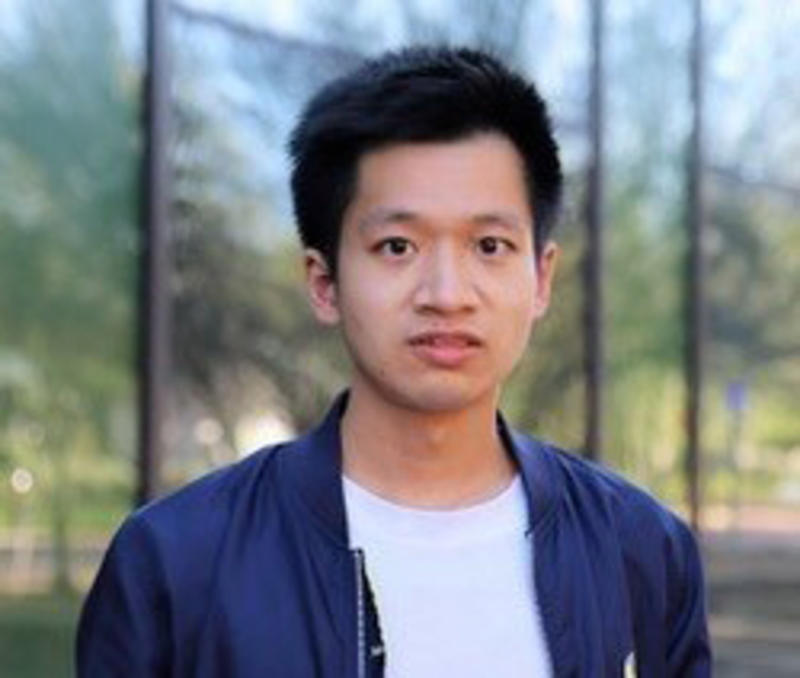# Yifan Chen### Abstract

Partial differential equations (PDEs) models are fundamental in today's applied and computational science. This talk presents a conceptually simple and algorithmically automatic framework for solving, learning, and uncertainty quantification of nonlinear PDEs. The framework assigns Gaussian processes (GPs) priors to the unknown functions and finite-dimensionalizes the PDE into a generally nonlinear combination of linear measurements of the GPs at sampled collocation points. Performing statistical inference or machine learning on these observed processes may then address the computational PDE problem. We will talk about some theoretical insights and numerical experiments of this GP-based method for a wide range of nonlinear PDE problems. We will also discuss a near-linear complexity sparse Cholesky factorization algorithm for kernel matrices with PDE measurements. With such, our approach is scalable to massive collocation points.

### Our speaker

Yifan Chen is a fourth year graduate student in Applied and Computational Mathematics at Caltech. He works with Thomas Y Hou, Houman Owhadi and Andrew Stuart. Yifan’s research interests are mathematical theories and efficient algorithms in scientific computing and scientific machine learning. Yifan received the Caltech Kortchak Scholarship.

To become a member of the Rough Path Interest Group, register here for free.